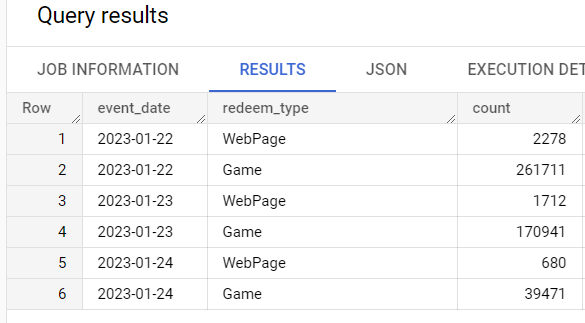# Percentage over a group by - window function sum over partition by

Hey there:)

I'm using Metabase open source version 0.45.1 on top of BQ.
I'm trying to create a percentage calculation for each group that I group by it.

I got this table and I'm trying to get for each redeem_type the percentage on each day.I could easily solve this using SQL-
SELECT event_date, redeem_type,count(1) count, sum(count(1)) over (partition by event_date) sum_per_day , count(1) / sum(count(1)) over (partition by event_date) as share

But I got questions about that-

1. Does window functions supported in the UI?
2. Is it possible to create this calculation, not through the SQL editor?